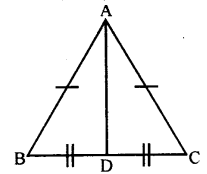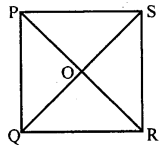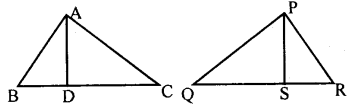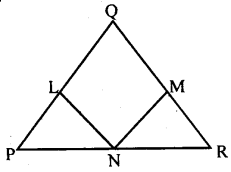# KSEEB Solutions for Class 8 Maths Chapter 11 Congruency of Triangles Ex 11.5

In this chapter, we provide KSEEB SSLC Class 8 Maths Chapter 11 Congruency of Triangles Ex 11.5 for English medium students, Which will very helpful for every student in their exams. Students can download the latest KSEEB SSLC Class 8 Maths Chapter 11 Congruency of Triangles Ex 11.5 pdf, free KSEEB SSLC Class 8 Maths Chapter 11 Congruency of Triangles Ex 11.5 pdf download. Now you will get step by step solution to each question.

### Karnataka State Syllabus Class 8 Maths Chapter 11 Congruency of Triangles Ex 11.5

Question 1.
In ∆ ABC, AC = AB and the altitude AD bisects BC prove that ∆ ADC = ∆ ADB.In ∆ ABD and ∆ ACD
AB = AC [data]
BD = DC [data]
∴ ∆ ABD = ∆ ACD [SSS postulate].

Question 2.
In a square PQRS, diagonals bisect each other at O. Prove that: ∆ POQ = ∆ QOR = ∆ ROS = ∆ SOP∆ POQ and ∆ QOR
PQ = QR [Sides of a square]
PO = OR [data]
OQ = OQ [Common side]
∴ ∆ POQ = ∆ QOR [SSS postulate] …i In AQOR and AROS QR = RS [Sides of a square]
QO = OS [data]
OR = OR [Common side]
∴ ∆ QOR = ∆ ROS [SSS postulate] …ii
In ∆ROS and ∆SOP
SR = SP [Sides of a square]
RO = OP [data]
OS = OS [Common side]
∴ ∆ ROS ≅ ∆SOP [SSSpostulate]…iii
From (i), (ii) and (iii)
∆POQ ≅ ∆QOR ≅ ∆ROS ≅ ∆SOP

Question 3.
In figure two sides AB, BC, and the median AD of ∆ABC are respectively equal to two sides PQ, QR, and median PS of ∆PQR. Prove that
Does if follow that triangles ABC and PQR are congruent.Ans
1. IN ∆ ADB and ∆ PSQ
AB = PQ [data]
BD = QS [BC = QR, D and S are the mid points]
∆ ABD ≅ ∆PQR [SSS postulate]
∠APB = ∠ PSQ [Corresponding sides]

2. ∆ ADC and ∆ PSR
AB = PQ [data]
DC = SR
[BC = QR, D and S are the mid points]
∠PSR = 180 -∠PSQ
∆ ABC ≅ ∆ PSR [SAS postulate]
Yes from the results of (i) and( ii)
∆ ABC = ∆ PQR

Question 4.
In ∆ PQR, PQ = QR, L, M, and N are the midpoint of the sides of PQ, QR, and RP respectively prove that LN = MNPQ = QR [data]
∠P = ∠R [Theorem l]
In ∆ PLN and RMN
PL = RM
[PQ = QR and L and M are the midpoints]
∠P = ∠R
PN = NR [N is the mid point of PR]
∴ ∆ PLN ≅ ∆RMN [SAS postulate]
∴ LN = MN [Corresponding sides]

All Chapter KSEEB Solutions For Class 8 maths

—————————————————————————–

All Subject KSEEB Solutions For Class 8

*************************************************

I think you got complete solutions for this chapter. If You have any queries regarding this chapter, please comment on the below section our subject teacher will answer you. We tried our best to give complete solutions so you got good marks in your exam.

If these solutions have helped you, you can also share kseebsolutionsfor.com to your friends.

Best of Luck!!